# 布洛赫球

## 单比特量子态

$\left|\psi\right> = a\left|0\right> + b\left|1\right>$

$\left|a\right|^2 + \left|b\right|^2 = 1$

$\begin{split}\left|0\right> = \begin{pmatrix} 1 \\ 0 \end{pmatrix}, \left|1\right> = \begin{pmatrix} 0 \\ 1 \end{pmatrix}\end{split}$

$\begin{split}\left|\psi\right> = \begin{pmatrix} a \\ b \end{pmatrix}\end{split}$

$\left|\psi\right> = \cos\left(\theta/2\right) \left|0\right> + e^{i\phi}\sin\left(\theta/2\right)\left|1\right>$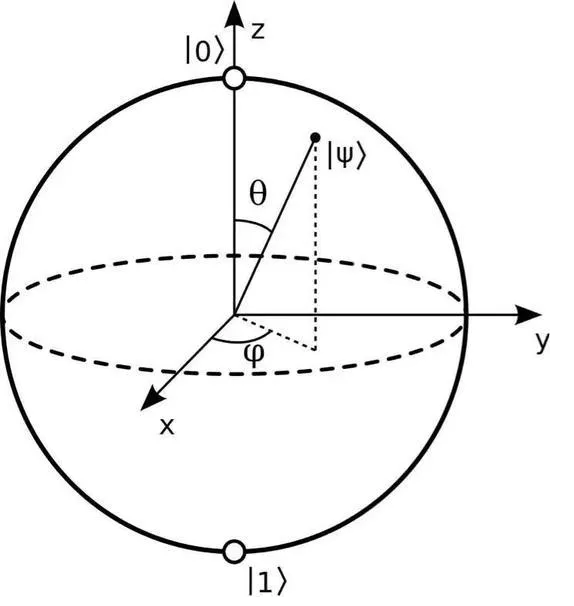## 导入相关模块

:

from mindquantum.core.circuit import Circuit
from mindquantum.core.gates import RX, RZ
from mindquantum.io.display import BlochScene


:

%matplotlib ipympl


## 搭建量子线路

:

circ = Circuit()               # 搭建制备任意单比特量子态的线路
circ += RX('theta').on(0)      # 通过RX门控制仰角
circ += RZ('phi').on(0)        # 通过RZ门控制方位角
circ.svg()

::

import numpy as np

state1 = circ.get_qs(pr={'theta': np.pi/4, 'phi': np.pi/4})
print(state1)

[0.85355339-0.35355339j 0.14644661-0.35355339j]


## 展示量子态

:

scene = BlochScene()                       # 创建布洛赫绘图场景
fig, ax = scene.create_scene()             # 初始化场景
state_obj1 = scene.add_state(ax, state1)   # 往场景中添加一个量子态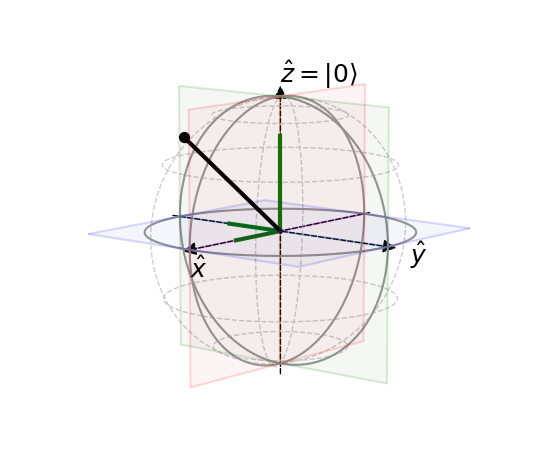:

scene = BlochScene('dark')                       # 创建布洛赫绘图场景
fig, ax = scene.create_scene()                   # 初始化场景
state_obj1 = scene.add_state(ax, state1)         # 往场景中添加一个量子态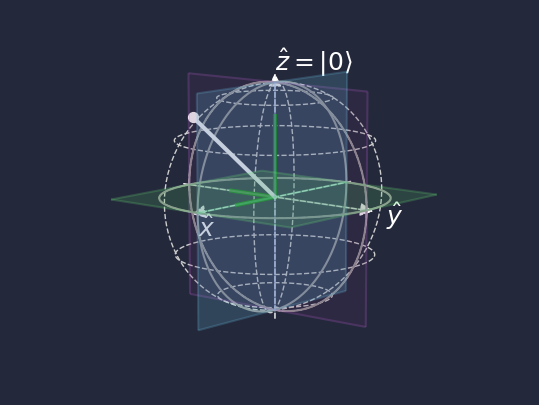## 展示量子态演化

:

t = np.linspace(0, 10, 500)
all_theta = 4 * np.sin(2 * t)
all_phi = 5 * np.cos(3 * t)
states = []
for theta, phi in zip(all_theta, all_phi):
states.append(circ.get_qs(pr={'theta': theta, 'phi': phi}))
states = np.array(states)


:

scene = BlochScene('dark')                          # 创建布洛赫绘图场景
fig, ax = scene.create_scene()                      # 初始化场景
state_obj = scene.add_state(ax, states)          # 往场景中添加一个量子态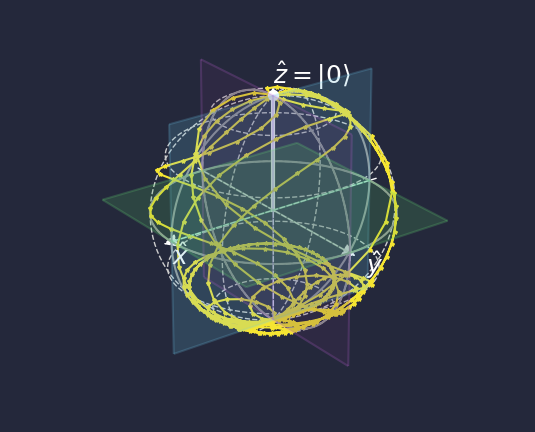:

anim = scene.animation(fig, ax, state_obj, states)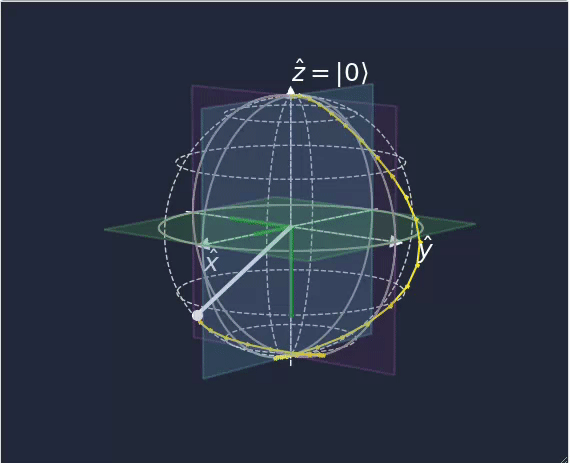:

from mindquantum.utils.show_info import InfoTable

InfoTable('mindquantum', 'scipy', 'numpy')

:

Software Version
mindquantum0.9.0
scipy1.10.1
numpy1.23.5
System Info
Python3.8.17
OSWindows AMD64
Memory8.39 GB